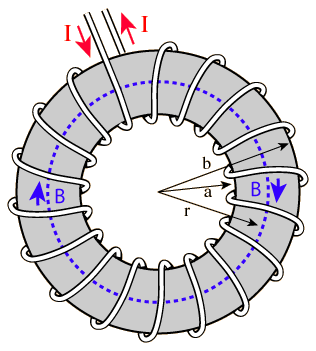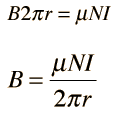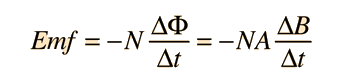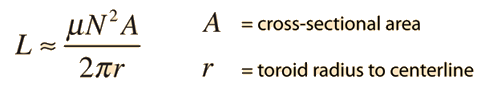# Approximate Inductance of a Toroid

Finding the magnetic field inside a toroid is a good example of the power of Ampere's law. The current enclosed by the dashed line is just the number of loops times the current in each loop. Amperes law then gives the magnetic field at the centerline of the toroid asThe inductance can be calculated in a manner similar to that for any coil of wire.

The application of Faraday's law to calculate the voltage induced in the toroid is of the formThis can be used with the magnetic field expression above to obtain an expression for the inductance.Toroidal radius r = cm with N = turns,

Coil radius = cm gives area A = cm2.

Relative permeability of the core k = ,

Then the inductance of the toroid is approximately

L = Henry = mH.

This is a single purpose calculation which gives you the inductance value when you make any change in the parameters.

Small inductors for electronics use may be made with air cores. For larger values of inductance and for transformers, iron is used as a core material. The relative permeability of magnetic iron is around 200.

This calculation is approximate because the magnetic field changes with the radius from the centerline of the toroid. Using the centerline value for magnetic field as an average introduces an error which is small if the toroid radius is much larger than the coil radius.

 Inductance of a solenoid
Index

Magnetic field concepts

 HyperPhysics***** Electricity and Magnetism R Nave
Go Back